# ISNUMBER Function Examples – Excel, VBA, & Google Sheets

This tutorial demonstrates how to use the Excel ISNUMBER Function in Excel to test if a cell is a number.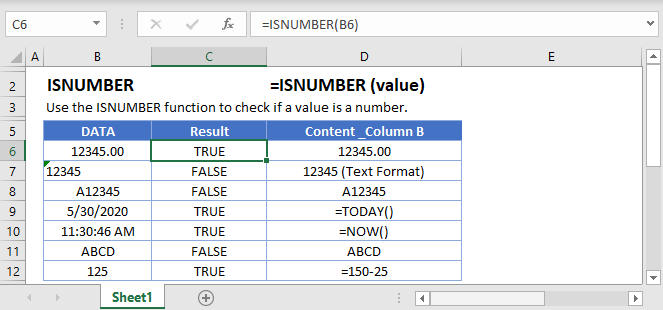## ISNUMBER Function Overview

The ISNUMBER Function Test if cell is a number. Returns TRUE or FALSE.

To use the ISNUMBER Excel Worksheet Function, select a cell and type: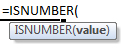(Notice how the formula inputs appear)

### ISNUMBER Function Syntax and Inputs:

value – The test value

## How to Use the ISNUMBER Function

The ISNUMBER Function tests if a cell is a numerical value. If yes, it returns TRUE, otherwise it returns FALSE.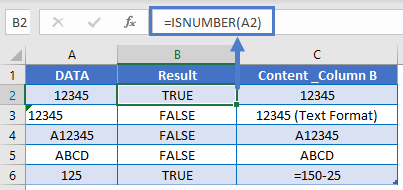#### Things to Know

• In Excel, Dates and Times are stored as numeric values. ISNUMBER will return TRUE when evaluating Dates and Times.
• Number stored as text will return FALSE.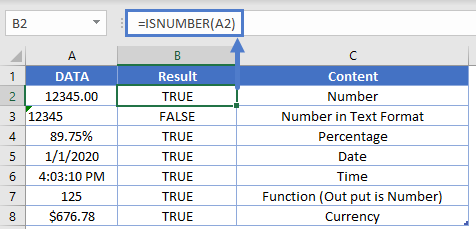### If Cell is Number Then

Often, you’ll want to combine an “IS” function, like ISNUMBER, with the IF Function. With the IF Function, instead of returning a simple TRUE or FALSE, you can output specific text or perform specific actions if a cell is a number or not.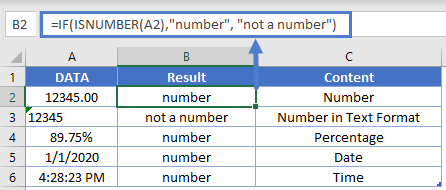### Check if Not Number

With the NOT Function, you can test if a cell is not a number.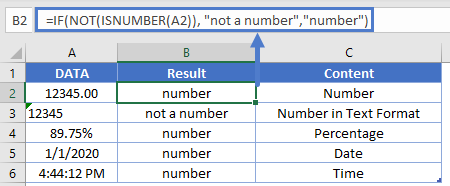## ISNUMBER & SEARCH Formula

One of the most useful features of the ISNUMBER Function is the ability to evaluate if certain functions return a numerical value. For example, the SEARCH Function searches a cell for text. If the text is found, it’s numerical position is returned. If the text is not found an error is returned. By using the ISNUMBER Function we can return TRUE / FALSE if the text is found.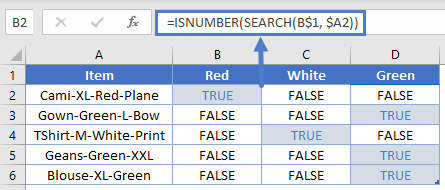### ISNUMBER & FIND – Case Sensitive

The FIND Function works exactly the same as the SEARCH Function, except the FIND Function is case sensitive. In other words, the FIND Function will return FALSE when searching for “string” in “STRING” because the cases do not match.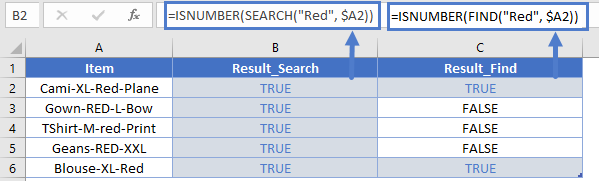### ISNUMBER & MATCH

The ISNUMBER Function can also be paired with the MATCH Function in a similar fashion. The MATCH Function searches for a value in a range of values. If the value is found, the function returns it’s numerical position. If it’s not found, it returns an error.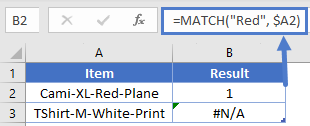## Validate Cell Input

One use of the ISNUMBER Function is to validate the contents of a cell. For example, you might have an input form that requires a numerical value. With the ISNUMBER Function you can test if the user entered a numerical value, alerting them if they did not.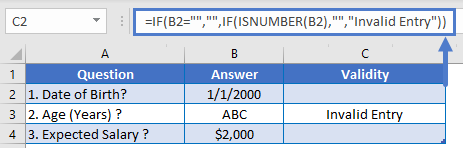### Data Validation – Force Text Entry

The above example was “weak” data validation; the user can ignore the warning message. Instead, we can use Data Validation to disallow non-numerical values.

Go to: Data Ribbon > Data Validation > Data Validation

and then select from one of these options: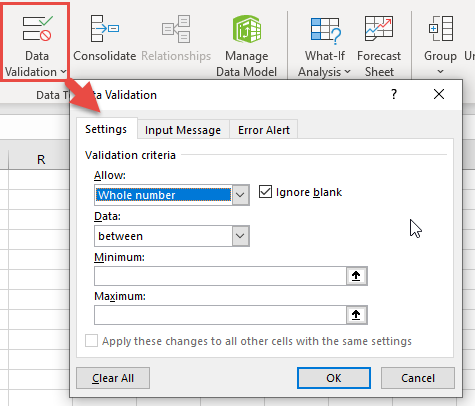## Test if Any Cell in Range is a Number

To test if any cell in a range of cells is a number, we can use the ISNUMBER Function along with the SUMPRODUCT Function.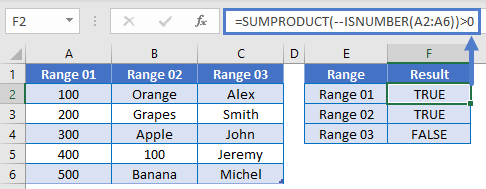### Highlight Cells That Contain Numbers

One last thing you can do with the ISNUMBER Function is highlight cells that contain text. We can do this by creating Conditional Formatting based on a rule.

1. Select all the cells that you want to check
2. Go to Home Ribbon > Conditional Formatting > New Rule
3. Select ‘Use a formula to determine which cells to format
4. Enter the formula =ISNUMBER(A2)
5. Click the Format button and choose your desired formatting
6. Click OK twice to quit and save.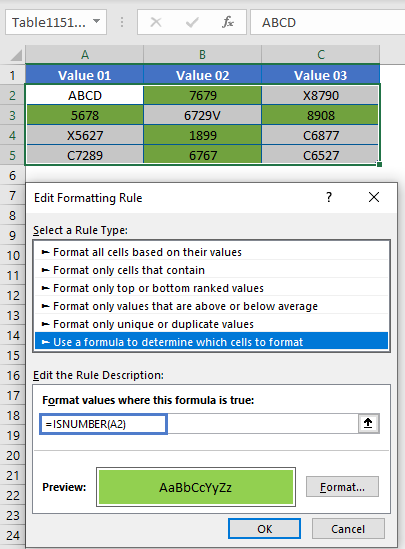### Other Logical Functions

Excel / Google Sheets contain many other logical functions to perform other logical tests. Here is a list:

IF / IS Functions
iferror
iserror
isna
iserr
isblank
isnumber
istext
isnontext
isformula
islogical
isref
iseven
isodd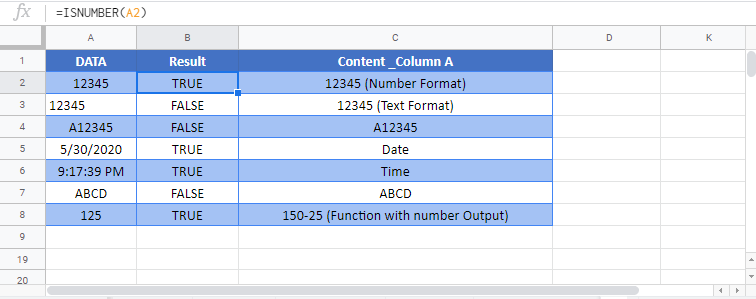`application.worksheetfunction.isnumber(value)`